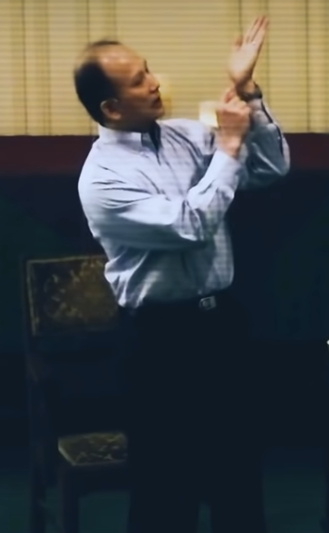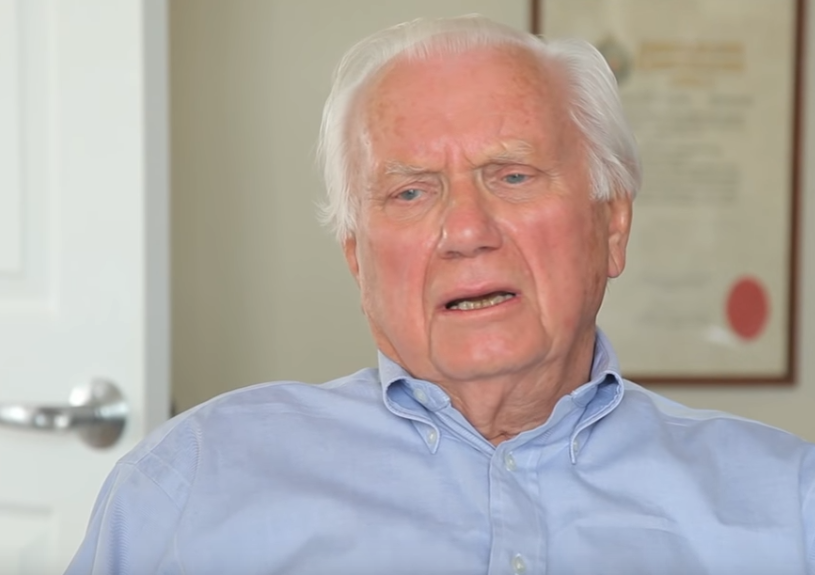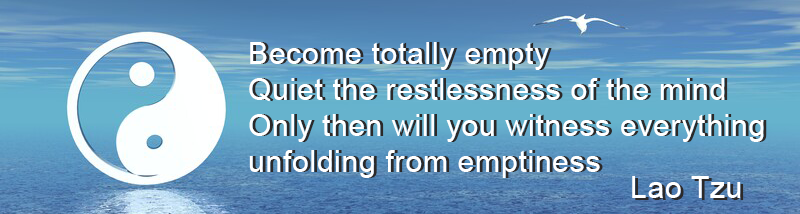Like   Tweet   Pin   +1   in/* styles */ Tips & resources on wellbeing & performance   ||   Issue 37, November 2021
 table div table+table+table div table{width:100%;padding:0}table div table+table+table div table img{width:96.23%;padding:0;float:none}table div table+table+table div table td{width:100%;padding:0 1.88% 18px}/* styles */In this issue there's an update of my work in aged care, a Tai Chi tip, Lao Tzu quote and two short video clips - one on the importance of visualisation, the other on meaning and purpose.

I hope you enjoy the quick read.

Regards
Chris Bennett
Chris Chi

 table div table+table+table+table+table div table{width:100%;padding:0}table div table+table+table+table+table div table img{width:96.23%;padding:0;float:none}table div table+table+table+table+table div table td{width:100%;padding:0 1.88% 18px}/* styles */## Back to 'Normal'I've recently received two bookings for my Chair Chi sessions for residents thanks to restrictions lifted in Aged Care centres

I'll still be required to wear a mask however I've run a few sessions with one so that should not be a problem.

I'm also organising an expression of interest to various other centres in Melbourne to arrange possible bookings for December and next year.

I'm looking forward to getting back into it as I enjoy working with residents..

Image courtesy of Ambreen Hasan via Unsplash.

 table div table+table+table+table+table+table+table+table div table{width:100%;padding:0}table div table+table+table+table+table+table+table+table div table img{width:96.23%;padding:0;float:none}table div table+table+table+table+table+table+table+table div table td{width:100%;padding:0 1.88% 18px}/* styles */## Tai Chi Tip

 /* styles */ When practicing a standing or sitting posture avoid slumping your body. Feel the body lift, from your tan tien (just below your belly button) towards the sky. Do not lift your shoulders.
 table div table+table+table+table+table+table+table+table+table+table+table div table{width:100%;padding:0}table div table+table+table+table+table+table+table+table+table+table+table div table img{width:96.23%;padding:0;float:none}table div table+table+table+table+table+table+table+table+table+table+table div table td{width:100%;padding:0 1.88% 18px}/* styles */## VisualisationVisualisation plays a crucial role in learning an internal art such as Tai Chi. In this clip Master Chunyi Lin gives one example of how to do this.

The technique he demonstrates is at 4.58 of this 8.01 clip though try and watch all of it to get a better understanding of the power of the mind.

If you decide to have a go let me know what it felt like.

 table div table+table+table+table+table+table+table+table+table+table+table+table+table+table div table{width:100%;padding:0}table div table+table+table+table+table+table+table+table+table+table+table+table+table+table div table img{width:96.23%;padding:0;float:none}table div table+table+table+table+table+table+table+table+table+table+table+table+table+table div table td{width:100%;padding:0 1.88% 18px}/* styles */## Meaning and PurposeEbert, 88 years old, talks about finding meaning and purpose in life as an elderly person.

His advice applies to anyone who lacks meaning and purpose in life. For some it's easy for others the search goes on.

 table div table+table+table+table+table+table+table+table+table+table+table+table+table+table+table+table+table div table{width:100%;padding:0}table div table+table+table+table+table+table+table+table+table+table+table+table+table+table+table+table+table div table img{width:96.23%;padding:0;float:none}table div table+table+table+table+table+table+table+table+table+table+table+table+table+table+table+table+table div table td{width:100%;padding:0 1.88% 18px}/* styles */table div table+table+table+table+table+table+table+table+table+table+table+table+table+table+table+table+table+table+table div table{width:100%;padding:0}table div table+table+table+table+table+table+table+table+table+table+table+table+table+table+table+table+table+table+table div table img{width:96.23%;padding:0;float:none}table div table+table+table+table+table+table+table+table+table+table+table+table+table+table+table+table+table+table+table div table td{width:100%;padding:0 1.88% 18px}/* styles *//* styles */
 table div table+table+table+table+table+table+table+table+table+table+table+table+table+table+table+table+table+table+table+table+table div table{width:100%;padding:0}table div table+table+table+table+table+table+table+table+table+table+table+table+table+table+table+table+table+table+table+table+table div table img{width:96.23%;padding:0;float:none}table div table+table+table+table+table+table+table+table+table+table+table+table+table+table+table+table+table+table+table+table+table div table td{width:100%;padding:0 1.88% 18px}/* styles *//* styles */